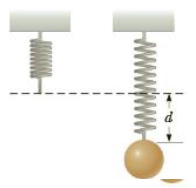Chapter 13, Problem 4P

Chapter
Section
Textbook Problem

A spring is hung from a ceiling, and an object attached to its lower end stretches the spring by a distance d = 5.00 cm from its unstretched position when the system is in equilibrium as in Figure P13.4. If the spring constant is 47.5 N/m, determine the mass of the object.To determine
The mass of the object which stretches the spring by 5 cm.

Explanation

Given information: The object stretches the spring by 5 cm. The force constant of the spring is 4.75N.m-1 .

The formula for force is,

F=kx

• F is the force
• k is force constant of the spring
• x is the displacement of the spring

It is equal to the force of gravity.

F=mg

• m is the mass of the object
• g is the acceleration due to gravity

Equate the two equations and re-arrange to get m.

m=kxg

Substitute 5 cm for x, 4.75 for k and 9

Still sussing out bartleby?

Check out a sample textbook solution.

See a sample solution

The Solution to Your Study Problems

Bartleby provides explanations to thousands of textbook problems written by our experts, many with advanced degrees!

Get Started

Find more solutions based on key concepts